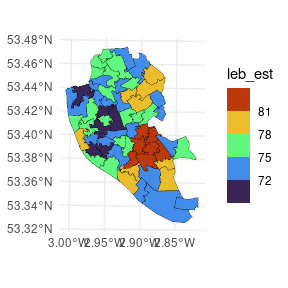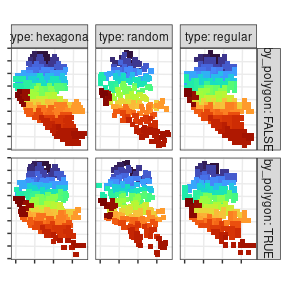# 1. Converting sf objects to spm

library(smile)
library(ggplot2)
library(sf)

Often, in an applied setting, it is desirable to change the spatial support of some variables in order to conduct either association or regression analysis. This package provides functions to deal with this problem under two different approaches, a model-based one and a (non-parametric) spatial interpolation.

The purpose of this vignette is to illustrate how to convert sf(Pebesma 2018) objects to objects support by the smile package. Besides these two packages, we are going to use the ggplot2(Wickham 2011) package for the data visualization.

This vignette is useful when the user wants to pursue the model-based approach. The methodology is based on the assumption that areal data (e.g., variables measured at census tracts) are composed by averages of a continuous underlying process (Gelfand, Zhu, and Carlin 2001; Moraga et al. 2017, @johnson2020dealing; Wilson and Wakefield 2020). In practice, there exists an identifiability problem when estimating some variance parameters, which can be seen either as small scale variation (nugget effect) or measurement errors. When fitting models, the users may chose to ignore the measurement error associated with the problem. Model fitting and prediction are explained in the vignette available on this link.

To illustrate how to convert sf polygons into spm objects, we are going to use the datasets provided by Johnson, Diggle, and Giorgi (2020). For this data, we have the Life Expectancy at Birth (LEB) and the Index of Multiple Deprivation (IMD) in Liverpool. These variables are observed at the Middle Super Output Areas (MSOA) and Lower Super Output Areas (LSOA), respectively. After loading our package, the datasets can be loaded by simply running the chunk of code below. Figure displays the LEB at the MSOA.

data(liv_lsoa) # loading the LSOA data

## workaround for compatibility with different PROJ versions
st_crs(liv_msoa) <-
st_crs(liv_msoa)$input st_crs(liv_lsoa) <- st_crs(liv_lsoa)$input
ggplot(data = liv_msoa,
aes(fill = leb_est)) +
geom_sf(color = "black",
lwd   = .1) +
scale_fill_viridis_b(option = "H") +
theme_minimal()LEB in Liverpool at the MSOA.

Now, suppose we are interested in estimating the LEB at the LSOA, so we will be able to conduct association analysis between LEB and IMD at the MSOA level. We assume, that the LEB, denoted $$Y(\cdot)$$, is driven by a stationary and isotropic continuous Gaussian process over the region of study, such that, the observed data at the $$i$$-th MSOA area (denoted $$A_i$$) is an average of this underlying process. If we knew the parameters and covariance function associated with this process, then the LEB at the $$i$$-th MSOA would be as follow $Y(A_i) = \frac{1}{\lvert A_i \rvert} \int_{A_i} Y(\mathbf{s}) \, {\rm d} \mathbf{s},$ where $$\lvert A_i \rvert$$ stands for the area associated with the region $$A_i$$.

Similarly, \begin{align*} {\rm E}[Y(A_i)] & = \frac{1}{\lvert A_i \rvert} \int_{A_i} {\rm E}[Y(\mathbf{s})] \, {\rm d} \mathbf{s} \\ & = \frac{1}{\lvert A_i \rvert} \int_{A_i} \mu \, {\rm d} \mathbf{s} \\ & = \mu, \end{align*} and \begin{align*} {\rm Cov}[Y(A_i), Y(A_j)] & = \frac{1}{\lvert A_i \rvert \lvert A_j \rvert} \int_{A_i \times A_j} {\rm Cov}[Y(\mathbf{s}, Y(\mathbf{s}')] \, {\rm d} \mathbf{s} \, {\rm d} \mathbf{s'} \\ & = \frac{1}{\lvert A_i \rvert \lvert A_j \rvert} \int_{A_i \times A_j} {\rm C}( \lVert \mathbf{s} - \mathbf{s}' \rVert ; \boldsymbol{\theta}) \, {\rm d} \mathbf{s} \, {\rm d} \mathbf{s'}, \end{align*} where $$\lVert \mathbf{s} - \mathbf{s}' \rVert$$ is the Euclidean distance between the coordinates $$\mathbf{s}$$ and $$\mathbf{s}'$$, and $${\rm C}( \lVert \mathbf{s} - \mathbf{s}' \rVert ; \boldsymbol{\theta})$$ is an isotropic covariance function depending on the parameter $$\boldsymbol{\theta}$$.

In practice, however, the integrals in the equation above are hard to be evaluated analytically. A common workaround is to evaluate them either numerically or by Monte Carlo integration (Gelfand, Zhu, and Carlin 2001). When using the function sf_to_spm, the parameter "type" controls the method of integration. The options are "regular" (or "hexagonal") for numerical integration, or "random" for Monte Carlo integration. Regardless of the "type" chosen, we have to generate a grid of points over the study region. When doing so, we may chose whether we want to approximate the integral within each area with the same precision or if we want the precision to vary according to the size of the polygon. This is controlled by the parameter "by_polygon", which is a boolean scalar. When set to TRUE, all the integrals will be estimated with the same precision, regardless of the size of the polygon. On the other hand, if this parameter is set to FALSE, the grid of points will be generated over the whole study region and, afterwards, the points will be attributed to the areas they are contained in. This way, larger polygons will contain more points and, thus, the respective integrals will have a smaller numerical error. Lastly, there exists a parameter called "npts". This parameter controls the number of points used to compute this integrals. We may either input a vector with the same length as the number of areas or a scalar. When inputting a scalar, this scalar will stand for the number of points over the whole city if by_polygon = FALSE and the number of points per polygon (area) otherwise.

If we wish to estimate the LEB in the LSOA areas, we will need to create a spm object associated with this variable, fit the model, and then compute the predictions. The chunk of code below shows how to convert the liv_msoa (of class sf) to a spm object. In this case, we are generating a grid of 1000 points over the whole city of Liverpool, then we will be attributing each of these points to the area they are contained in. Also, the "poly_ids" argument is a string indicating the variable in the liv_msoa dataset that contains the unique identifier associated with each area. The argument "var_ids" is a string as well but this indicates the “response variable”. That is, the variable that for which we will be interested in fitting a model to.

msoa_spm <-
sf_to_spm(sf_obj = liv_msoa, n_pts = 1000,
type = "regular", by_polygon = FALSE,
poly_ids = "msoa11cd", var_ids = "leb_est")

Finally, the Figure below displays the grids associated with each of the possible combinations of the parameters type and by_polygon when calling the sf_to_spm function.For details on fitting models and making precitions, see this vignette.